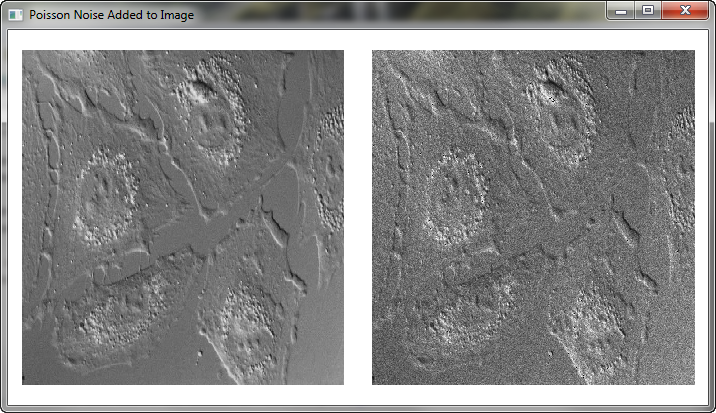Adding Poisson Noise to an ImageQUESTION: What is the best way to add random Poisson noise to an image in IDL? I would like to add five percent random noise to my image.ANSWER: Yes, adding random Poisson noise to an image is a little harder than just adding random uniform noise to an image, since you have to compute the Poisson random deviate for each value in the image. (The fact that the Poisson keyword to RandomU only accepts a scalar Poisson mean is what makes this step more difficult.)

Wayne Landsman, curator of the NASA IDL Astronomy Library, recommends using the POIDEV routine from that library, which makes the process exceedingly easy.

IDL> image = image + 0.5 * PoiDev(image)

However, you can also write your own function in IDL to do this, as shown in the code below. The function is named AddPoissonNoise, and it is adapted from a program offered by Wayne Landsman and modified by David Platten in a 12 April 2013 IDL newsgroup article on this subject.

SetDefaultValue, image, cgDemoData(20)
SetDefaultValue, percentNoise, 5.0
h = cgHistogram(image, MIN=0, REVERSE_INDICES=ri)
noiseImage = image
FOR j=0,N_Elements(h)-1 DO BEGIN
currentMean = j
indices = ReverseIndices(ri, j, COUNT=count)
IF count GT 0 THEN BEGIN
noiseImage[indices] = RandomU(seed, count, POISSON=currentMean)
newMean = SqRt(currentMean) / (percentNoise/100.0)

; Adjust the standard deviation to the required level.
noiseImage[indices] += newMean - currentMean

; Now adjust it back to starting the mean, but with noise added.
noiseImage[indices] *= currentMean / newMean
ENDIF
ENDFOR
RETURN, noiseImage
END

You can test the function like this, in which 15% noise is added to the image.

image = cgDemoData(20)
seed = -3L ; Only so that the example here matches your result!
Print, "Mean and standard deviation of original image is: " \$
+ StrTrim(Mean(image),2) + ", " + StrTrim(StdDev(image),2)
Print, "Mean and standard deviation of noisy image is: " \$
+ StrTrim(Mean(noisyImage ),2) + ", " + StrTrim(StdDev(noisyImage),2)
!P.Multi=[0,2,1]
cgDisplay, 700, 375, /Free, Title='Poisson Noise Added to Image'
cgImage, image, MultiMargin=2
cgImage, noisyImage, MultiMargin=2
!P.Multi = 0

Executing these commands produces the following output, and the image plot shown in the figure below.

Mean and standard deviation of original image is: 115.418, 21.1413
Mean and standard deviation of noisy image is: 113.930, 27.4261Original image on the left and image with 15% noise added on the right.